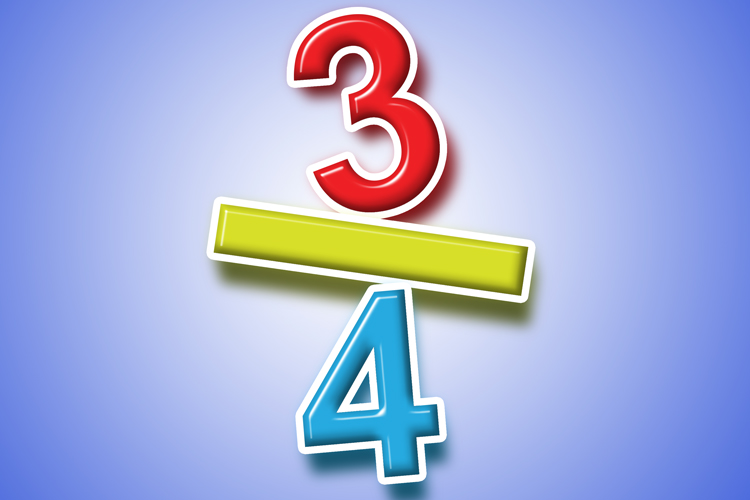# Fractions

## Numerator and denominator

The numerator is the top part of the fraction, while the denominator is the bottom part of the fraction.

NUmerator = Up (U for up)
Denominator = Down (D for Down)

AndThe denominator is dominated by the number on the top.

Example 1Example 2Here 3 is the numerator and 4 is the denominator.

Example 3Here 17 is the numerator and 6 is the denominator.